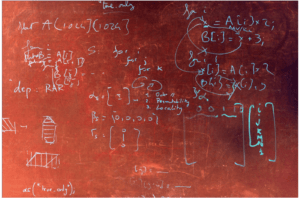# Combinatorial Multigrid for Lattice Quantum ChromodynamicsLattice Quantum Chromodynamics (LQCD) is a numerical approach to understanding the strong nuclear force, in which space and time are discretized into a 4D lattice. Calculating the quark propagator involves inverting sparse, roughly symmetric and diagonally dominant matrices with trillions of entries…

As an intern at Reservoir Labs, I worked on speeding up this computationally expensive inversion process. I implemented a Combinatorial Multigrid for preconditioning these matrices, and assessed the effectiveness of various fill-reduction techniques.